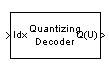# Quantizing Decoder

Decode quantization index according to codebook

## Library

Source Coding

•## Description

The Quantizing Decoder block converts quantization indices to the corresponding codebook values. The Quantization codebook parameter, a vector of length N, prescribes the possible output values. If the input is an integer k between 0 and N-1, then the output is the (k+1)st element of Quantization codebook.

The input must be a discrete-time signal. This block processes each vector element independently. For information about the data types each block port supports, see the Supported Data Type table on this page.

### Note

The Quantizing Encoder block also uses a Quantization codebook parameter. The first output of that block corresponds to the input of Quantizing Decoder, while the second output of that block corresponds to the output of Quantizing Decoder.

## Parameters

Quantization codebook

A real vector that prescribes the output value corresponding to each nonnegative integer of the input.

Quantized output data type

Select the output data type.

## Supported Data Type

PortSupported Data Types
Idx
• Double-precision floating point

• Single-precision floating point

• 8-, 16-, and 32-bit signed integers

• 8-, 16-, and 32-bit unsigned integers

Q(U)
• Double-precision floating point

• Single-precision floating point

## Pair Block

Quantizing Encoder